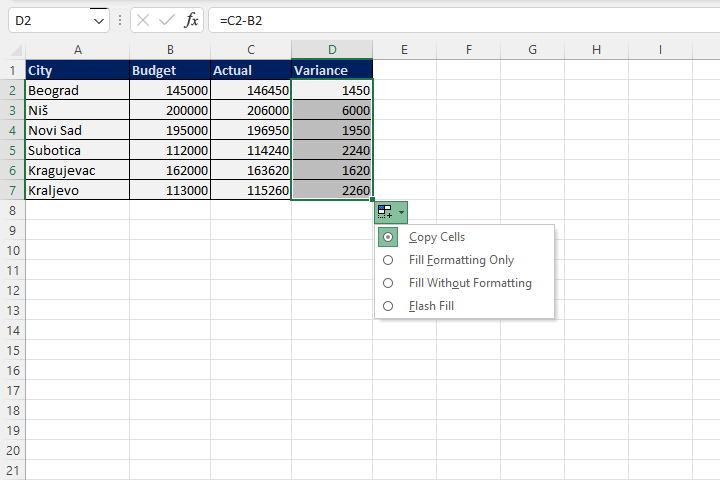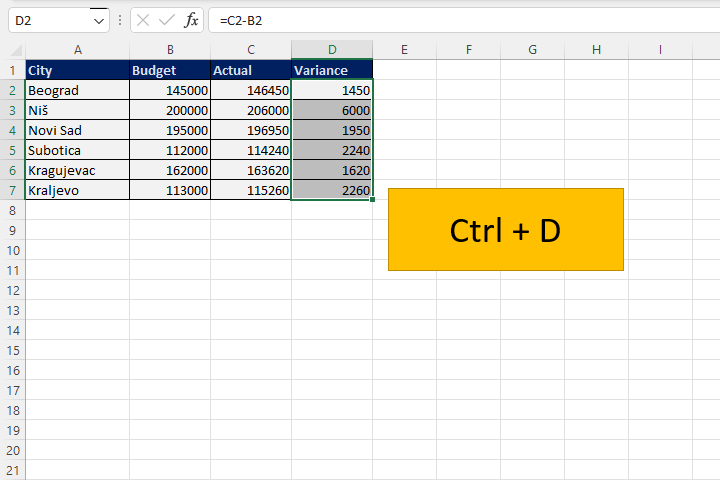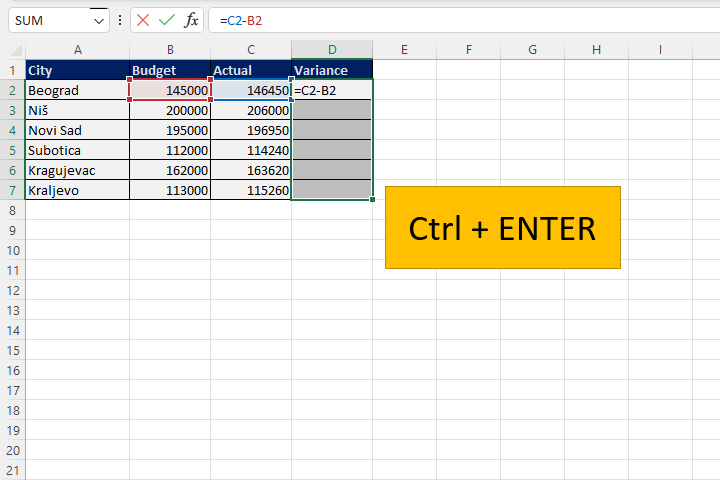# Copying formulas

There are several ways to multiply Excel formulas: by simply copying, creating a series that multiplies values, using a variant of the Paste Special option that copies formulas… However, in everyday work, shortcuts will mean a lot to you and they will make you more productive. In the text that follows, you will find out more about such shortcuts…

First, let’s show the traditional methods. There is a table in which you can see sales by city, namely: Budget, Actual and Variance. We calculate the variance as the difference between Actual and Budget. If we enter a formula that calculates the difference between C2 and B2 in cell D2, and then double-click on the square in its lower right corner, the formula will be copied to the bottom of the table by automatically applying the Fill Series option. The only thing left to do is to confirm copying by selecting the Copy option.Alternatively, we can use one of the shortcuts for Fill Series in the given direction. Copying the contents of a cell, in this case a formula, is achieved by selecting the cell with the formula and all the rest in the column and then pressing the CTRL+D(own) key combination. If the table had been organized differently, we could have used CTRL+R(ight), CTRL+U(p) or CTRL+L(eft).Finally, if we haven’t entered the formula yet, it is possible to select the entire column or row of the table in which the formula is entered, and after entering the expression in the Formula Box, one should press the CTRL+ENTER key combination instead of the ENTER. This way, the formula is entered in the range of selected cells.